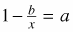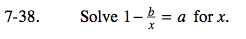Home > A2C > Chapter 7 > Lesson 7.1.3 > Problem7-38

7-38.

Solvefor x. Homework Help ✎Subtract 1 from both sides.

$-\frac{\textit{b}}{\textit{x}}=\textit{a}-1$

Now multiply both sides by x and then divide both sides by (a − 1).

$\textit{x}=\frac{\textit{b}}{1-\textit{a}}$